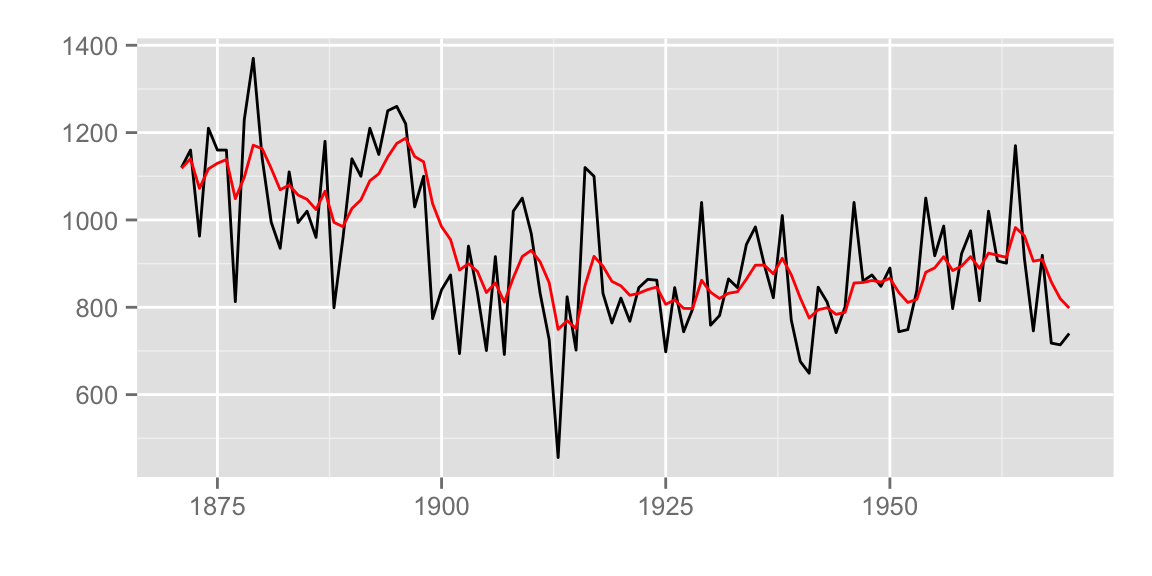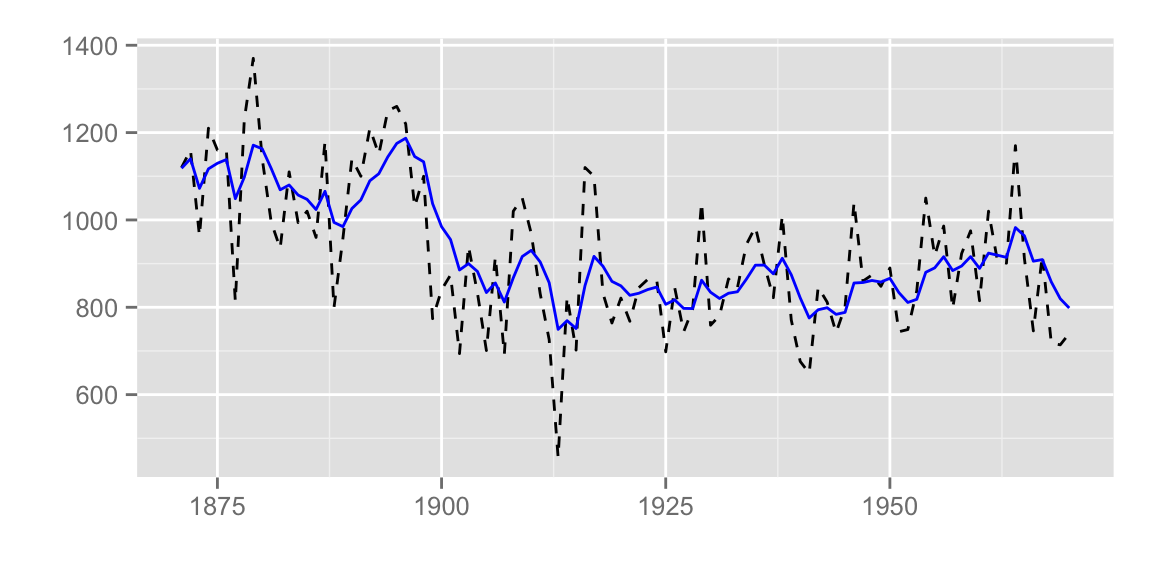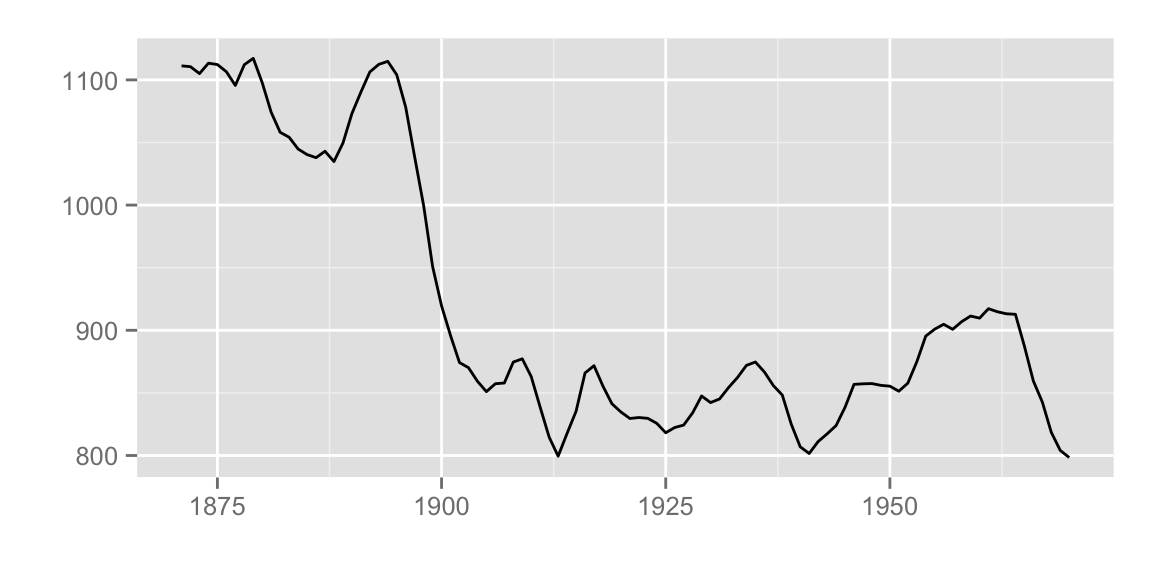This document explains state space time series related plotting using `ggplot2` and `ggfortify`.

# Installation

First, install `ggfortify` from github.

``````library(devtools)
install_github('sinhrks/ggfortify')``````

# Plotting with `{dlm}` package

`ggfortify` let `ggplot2` know how to interpret `{dlm}` instances. After loading `ggfortify`, you can use `ggplot2::autoplot` to plot them.

``````library(ggplot2)
library(ggfortify)``````

`autoplot` draws both original and fitted time series, because `dlm::dlmFilter` contains them.

``````library(dlm)
form <- function(theta){
dlmModPoly(order = 1, dV = exp(theta), dW = exp(theta))
}

model <- form(dlmMLE(Nile, parm = c(1, 1), form)\$par)
filtered <- dlmFilter(Nile, model)

autoplot(filtered)``````You can specify some options to change how plot looks, such as `ts.linetype` and `fitted.colour`. Use `help(autoplot.tsmodel)` (or `help(autoplot.*)` for any other objects) to check available options.

``autoplot(filtered, ts.linetype = 'dashed', fitted.colour = 'blue')```ggfortify` can plot `dlm::dlmSmooth` instance which returns a `list` instance using using class inference. Note that only smoothed result is plotted because `dlm::dlmSmooth` doesn’t contain original `ts`.

``````smoothed <- dlmSmooth(filtered)
class(smoothed)``````
``##  "list"``
``autoplot(smoothed)``To plot original `ts`, filtered result and smoothed result, you can use`autoplot` as below. When `autoplot` accepts `ggplot` instance via `p` option, continuous plot is drawn on the passed `ggplot`.

``````p <- autoplot(filtered)
autoplot(smoothed, ts.colour = 'blue', p = p)``````